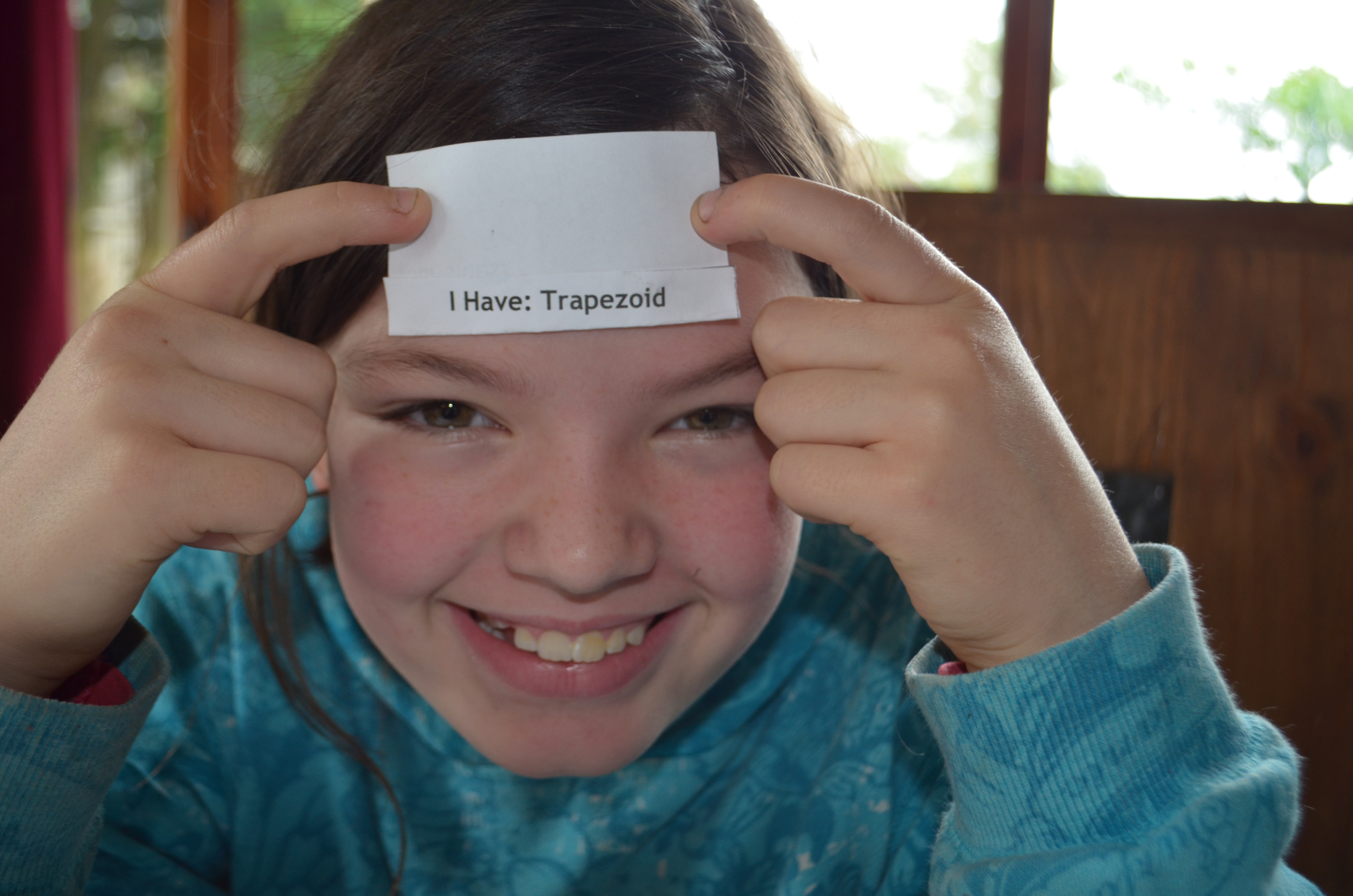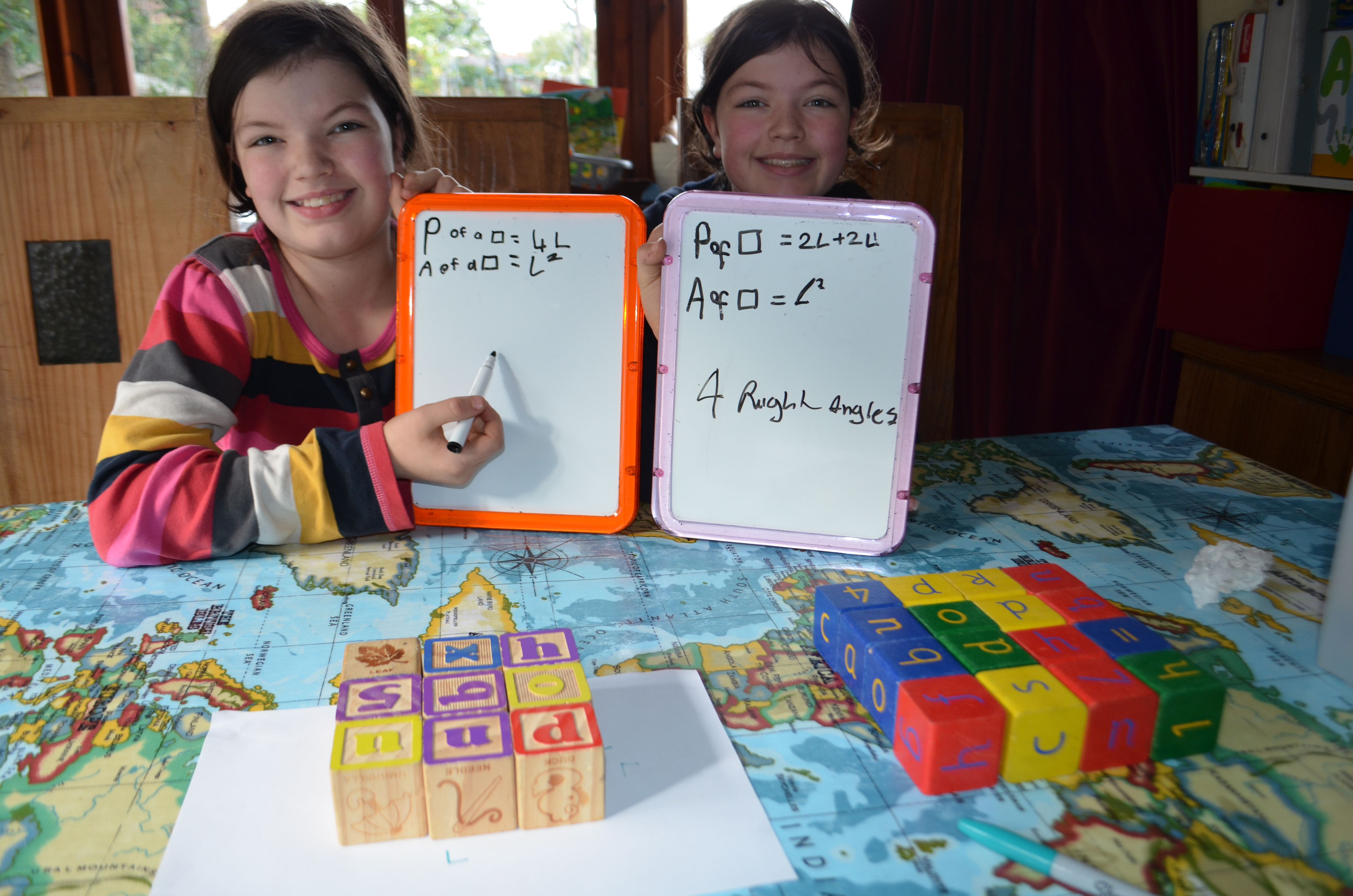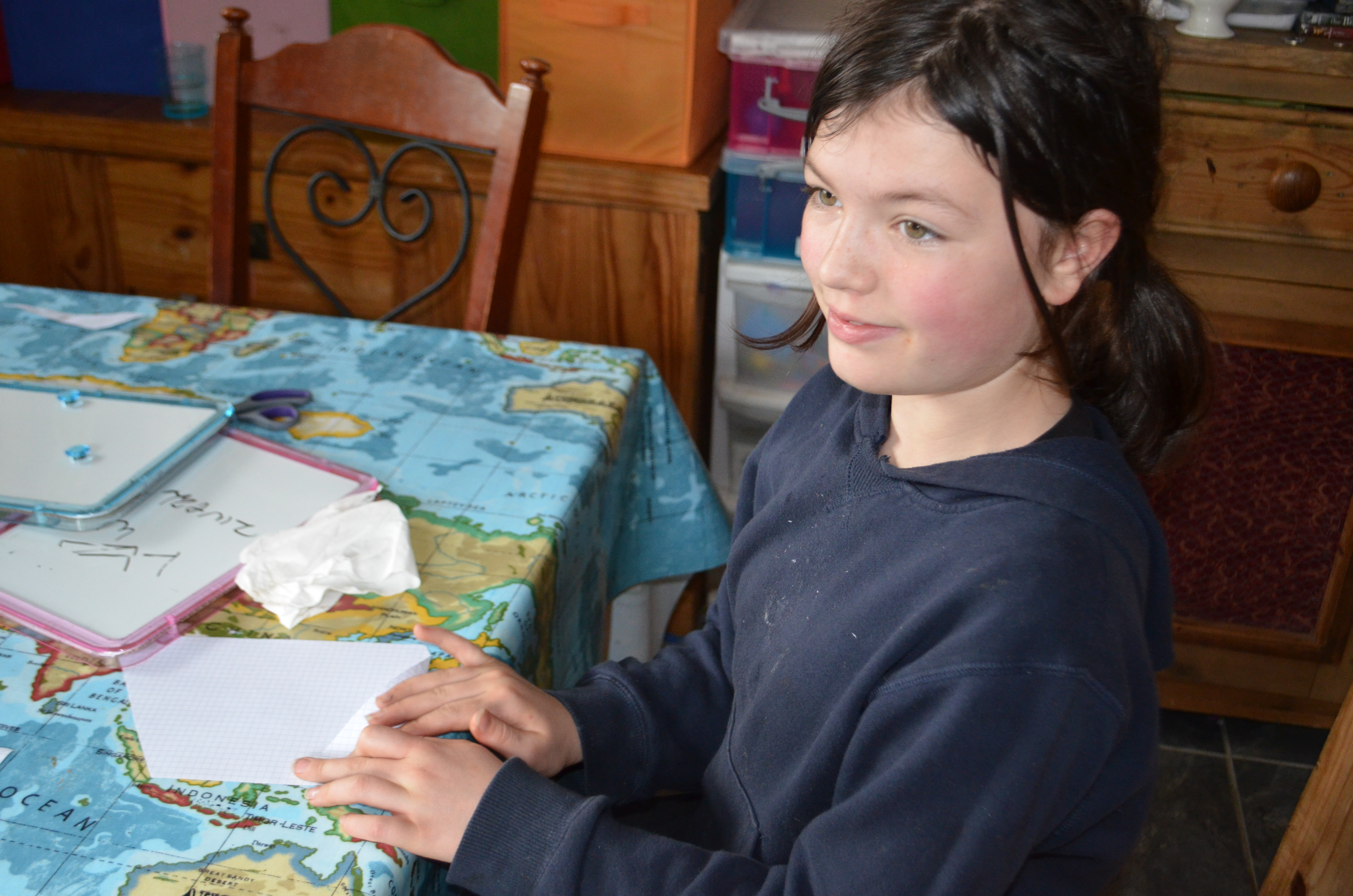# Helping a Struggling Maths Student: Quadrilaterals – Part Two

Discovery goals this week:

• Revise the quadrilaterals of last week
• Go over the nets of a cube ensuring each of the 11 nets are found
• Discover the formulae for the perimeter of all the quadrilaterals which are parallelograms and be able to explain why the formulae work
• Discover the formulae for the area of the quadrilaterals that are parallelograms and be able to explain why they work with these shapes but not the deltoid or trapezium

In order to practice the vocab we learnt last week and introduce a few more words I played a game whereby I gave them each a card with the name of each quadrilateral and I described one to them using mathematical language.  Then I asked who I was.  They had to choose the card which best fitted my description.  I wanted to introduce the word congruent to their list of vocal, used to describe two lines or shapes that are exactly the same in size and shape.

Next, the girls held an unknown quadrilateral name up on their head and had to ask questions to find out who they were:Each day I had them do a five minute exercise on the computer to make up the different quadrilaterals they had learnt.  This was easy, fun and great for revising the properties of each.

Last week the children had played about with magnetix to discover the nets for a cube.  They had found 6.  As there are 11 I gave them this exercise to do.  I was interested to see whether they would have sufficient understanding to visualise the nets they had missed.  I asked them first to try without any manipulatives but if they were struggling they were free to use anything that would be helpful.  I suggested marshmallows and sticks might be fun!

I brought out the big play blocks and had them build two squares of different sizes.  From here we discussed how to find the lengths, perimeter and area of the shape.  They have done this before and I knew they would find this fairly easy.  We discussed units and the types of units we could use to describe the measurements I had asked for.  In the absence of a ruler, they chose blocks and blocks squared as their units.  I then asked them to work out the formula.  This was interesting because they have done this many, many times before and I was quite surprised they had completely forgotten the formula.  They did know how to do it, however and working their way back from their result they came up with the following formulae for finding the perimeter and area of any square:We did the same exercise with rectangles and then I asked them to figure out why it works.  They thought it had something to do with the straight lines until I pointed out that triangles had straight lines but that wasn’t how the perimeter and area of a triangle was found.  Then one of them shouted out that it was the four right angles.  Yay!

Next we investigating the perimeter and area of a parallelogram and rhombus (a type of parallelogram).  I gave them two cardboard cut outs each of a rhombus and parallelogram and two identical ones cut out of squared paper.  I asked them to give me some methods to work out the perimeter and area of the two shapes.  For the perimeter they said they could measure using a ruler or a piece of string and then measure the piece of string.  The area wasn’t so simple, although L10 did say they could count the squares which was accurate if not a little laborious.  I asked them whether the formula for the rectangle and square would or wouldn’t work and why or why not.  They thought not and after some prodding they mentioned the lack of square corners.

It was at this point I gave them a pair of scissors each and told them to figure out the correct formula!  They looked at me with stunned suspicion to which I had to laugh!  T11 was now with us and I suggested they look at what they knew to be true from the day before (formula for area of square/rectangle = length x width) and try to find a way to use that formula with the rhombus and the parallelogram.  L10 was away!  This is the girl who cried everyday over her maths and she is now simply getting it because she can touch and therefore visualise it:L10 started off by attempting to fold her shapes to create a four right angled shape, which she did by snipping off the ends. She was unsure what to do with the two resulting triangles though.

It was T11 who figured out that you placed the two triangles together to make a second rectangle:Putting together all the cut out pieces of the parallelogram the children found they could make a rectangle and therefore apply the formula they already knew

Probably a bit unnecessarily, I asked them to demonstrate that the cut out rectangle had the same area as the parallelogram, which they did easily:The orange parallelogram is under the cut out squared paper one. It can be seen that they cover exactly the same area

Now for the tricky part.  They understood that the same formula could be used, but when I asked where the width was on a parallelogram they all thought it was the slanted line.  We went back to their cut out versions and found that the width of the cut out rectangle was actually the height of the parallelogram i.e. the distance between the top and bottom line.  I wrote out on the board their narration of what was going on:They were perplexed, because obviously the formula for a parallelogram is slightly different even though the maths is the same: area of a parallelogram = height x length  I simply reminded them that letters were only representational of numerical values and not to worry too much.  The important thing was to understand the dimensional maths of each shape rather than just learning a set of formulae.

I placed examples of all the quadrilaterals and asked them to put into one pile those for which they had a formula for the area and perimeter and in another pile those they had no formula for.  We then discussed what the difference was between the two piles.  The children were able to state that the pile with the formulae were all parallelograms whilst the other pile were not.  I told them we would be revisiting the second pile after we had investigated triangles as there is a link between the areas of quadrilaterals which are not parallelograms, and triangles.

I had some questions from a year 7 paper (grade 6) which I gave the girls to check their understanding.  T11 flew through them, whilst L10 and C10 plodded through.  They both got stuck on one when the wording threw them.  In this question they were given the area and perimeter of a rectangle and had to work out the length and width.  Once I had demonstrated how to work it out they were away!  I wrote out a few more to check they understood, which they did.  What struck me most about the girls working through these problems was their obvious confidence.  Even when they struggled there were no tears just a willingness to listen and understand and then just get on with it.  They no longer have maths anxiety.

Next week we will be investigating square numbers, and continuing with area and perimeter.